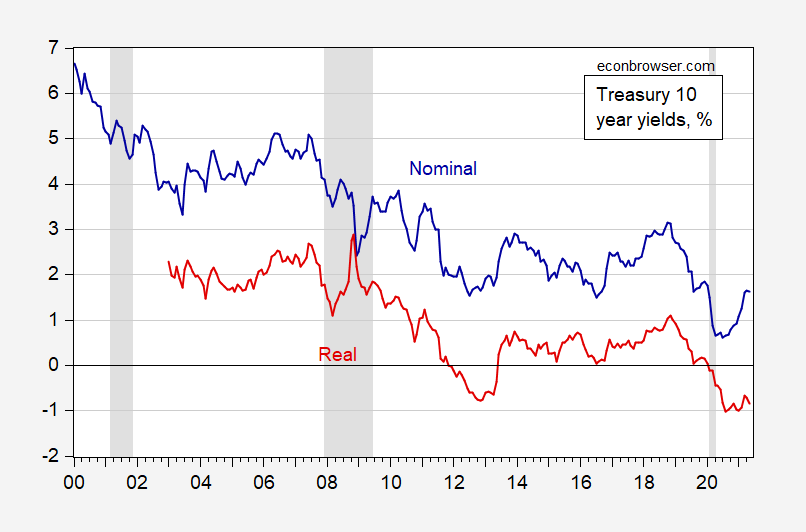## Debt Dynamics, And The Real Interest Rate

Debt-to-GDP dynamics are described by this expression.

(2)   dt-dt-1 = [(rt-gt)/(1+gt)]× dt-1 – pt

Where d is the debt to GDP ratio, r is the real (inflation-adjusted) interest rate, g is the growth rate of real GDP, and p is the primary (noninterest) surplus to GDP ratio. In words, the debt to GDP ratio rises when the real interest rate-growth rate gap is sufficiently positive, or the primary deficit is sufficiently large.

May 10-year TIPS was -0.85%. As of yesterday, that rate was -0.84% and -0.05% for 30-year.Figure 1: Nominal 10 year Treasury yield (blue), 10 year TIPS (red), both in %. NBER recession dates shaded gray; latest recession assumed to end 2020M04. Source: Federal Reserve via FRED, Treasury, and NBER.

Disclosure: None.

How did you like this article? Let us know so we can better customize your reading experience.

#### CommentsLeave a comment to automatically be entered into our contest to win a free Echo Show.